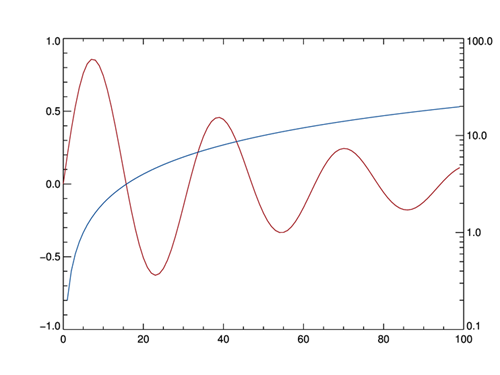QUESTION: What is the best way to add a second, logarithmic axis to my line plot?

ANSWER: An additional axis can be added to a plot with the cgAxis command. Be sure you set the Save keyword when you use the command, so that the subsequent overplotting of data will use the proper axis scaling.

Here is IDL code that plots a damped sine curve on a normal, linear Y axis and logarithmic data on a second, logarithmic axis. Click here to download a more complete code example.```   x = Findgen(100)
theta = x/5
curve = Sin(x/5) / Exp(x/50)

; Plot the damped sine curve. Suppress drawing the right-hand Y axis.
cgPlot, curve, YStyle=8, Color='red7'

; Draw a second, logarithmic axis on the right-hand side of the plot.
cgAxis, YAxis=1, YLog=1, YRange=[0.1, 100], /Save

; Overplot the logarithmic data.
cgOplot, theta, Color='blu7'
```

Here is an illustration of the plot produced by the IDL code above.